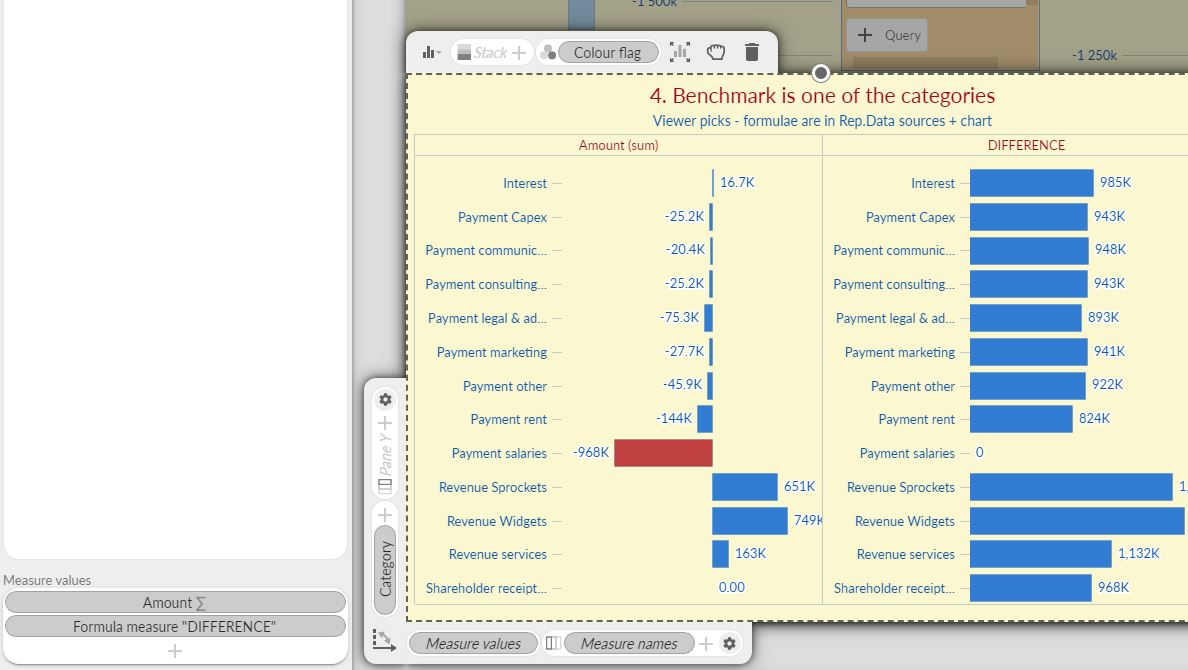# Dynamic benchmarking in Omniscope

Modified on Wed, 18 Nov 2020 at 11:56 AM

Benchmarking and targets can be visualised in different ways against the data values to help you keep track of your goals.

Here are some methods you can apply in Omniscope.

First scenario is that you have a single fixed value against which you compare various periods or categories in your dataset.

a) You can achieve this by adding a Formula measure to your chart containing the target value (e.g. 100,000) and combine it with other measures in the chart - here a Bar view.

Split field is [Category], measure [Amount] and the line measure is f(x)=100,000.

In this scenario, with every data update, the bar values will change, but the target will remain the same.

There are options to set up dynamic benchmarking, where the comparisons will respond to changes in the data or give control to the report viewer to decide on either the arbitrary benchmark number or an entity to be used for comparisons (e.g. compare the latest sales of all shops against a chosen shop).

b) target is a fixed number picked by the report viewer, controlled by a variable .

Add a numeric variable with slider control in the Report Data Sources section (under the 3-dot menu), then add in the Bar chart a Line measure formula = [Benchmark value]

(pick a variable in the formula builder)

c) different target for each category - for example factories with different capacity. Each one will have a target in line with their average/total production.

Use a Bar's Line formula measure with syntax like

SUBSET_SUM([Amount]) * 1.1

(to add 10% on top of the sum for each category, as per image. Because the chart is splitting the data into multiple 'buckets' this formula will be applied to each split/subset category data points)d) benchmark is a value based on calculation including all values, for example Industry mean.

Result can be calculated in the Report Data sources, and this field (with Mean function!) can be the Line measure in our Bar chart settings.

Result will respond to data changes, so our industry mean will reflect changes in performance of all players.

Formula field in the Report data sources will look like

[Industry average] = SUBSET_MEAN([Amount])

Because we have an Aggregation block upstream from this calculation, mean is calculated per aggregated category (e.g.[Company] , rather than on a transactional level.Note. By adding multiple formulas in the Report Data Sources area in the above scenario, we will enable our calculations to be sensitive to the exposed filters, so that report viewer can then easily exclude some periods or categories from the calculations.

Formula fields calculated here are available to all charts in this particular Report.

Important: For the Query in this chain to have an effect and influence the calculations downstream, the filter device exposed in the dashboard must contain the relevant Source 1 fields.e)

Let's say we wish to add a Bar chart that will contain 2 paned views - one that is comparing absolute [Amounts] for different categories in our accounting, the other pane dynamically calculating the difference between each bar category value and the chosen target.

In the Report Data sources, we'll add a variable, which is a category choice, containing the names of existing category values in our field, then several dynamic formulas:

IF(

[Category choice]='Interest',

SUBSET_SUM([Amount],subset([Category],'Interest')),

[Category choice]='Payment communications',

SUBSET_SUM([Amount],subset([Category],'Payment communications')),

[Category choice]='Payment salaries',

SUBSET_SUM([Amount],

subset([Category],'Payment salaries')),

null)

#our benchmark value is [Amount] sum for the category picked via the variable [Category choice]

Colour flag = IF([Benchmark category]=[Category],'red','blue')

#colouring in Omniscope will pick simple colour names in cells and colour the bars red or blue, depending if the Category value on that row matches the variable category choice.

We could add here a simple deduction formula to calculate the difference between each category's Amount sum and the Benchmark (chosen category's Amount value), but we can also do it in the Bar view, using the Formula measure:

SUBSET_SUM([Amount])

-

SUBSET_MEAN([Benchmark value]) (using mean to avoid duplication - benchmark value is repeated in all cells in that field)You could also perform similar type of analysis using the Bullet chart, as it has a setting for the Target field, which could be

• a field value (with a sum/mean or another function applied),
• a chart-specific calculated Formula measure
• a variable - here the same one we used in the Bar chart

In the Bullet visualisation you can also create 'zones' for good/expected/bad performance.

If all this is not perfectly clear - YOU CAN FIND ALL THE EXAMPLES in the ioz file attached below.